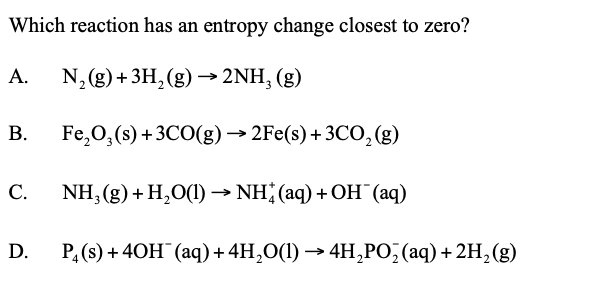# Entropy question IB chemistry

answer is B, I chose C. can someone explain? i thought b is wrong as it would be close to zero but negativeHi
In order to find entropy change, the reaction must be homogenous, which means the state of products and reactants should be in the same state: like the reaction A.

Heterogenous reactions would produce somewhat deviated answers if we find the difference in moles between products and reactants, so, we can simply remove C & D.

What we left is A & D,
D also is homogenous since solid & gas produce solid & gas.

When we differ the moles ( total moles of products - total moles of reactants)
In A, we get 2 - 4 = -2
In D, 2 mol of solid in product - 1 mol of solid in reactant = 1 mol difference
3 mol of gas in product - 3 mol of gas in reactant = 0
So, Reaction A has entropy change closest to zero!!

hope this helps